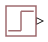# PS Step

Generate physical signal shaped as step function

Since R2021a

•Libraries:
Simscape / Foundation Library / Physical Signals / Sources

## Description

The PS Step block generates a physical signal that starts at a specified initial value and changes instantaneously to a new value at a specified time. The Step time, Initial value, and Final value parameters determine the characteristics of the output signal:

• The Step time parameter determines when the signal value changes from Initial value to Final value.

• The Initial value and Final value parameter values determine whether the output signal is a scalar, vector, or matrix. If one of these parameters is a vector or a matrix, the other must be a vector or a matrix of the same size or a scalar. The output signal is then also a vector or a matrix of the same size.

• The Initial value and Final value parameters must have commensurate units. The unit at the output port is determined by unit propagation rules. If the two parameters have the same units, then the output signal unit is also the same. If the parameter units are different, then the unit of the output signal is the base unit commensurate with the units of the parameters. For more information, see Physical Signal Unit Propagation.

## Ports

### Output

expand all

Output physical signal. The signal size matches the size of Initial value and Final value parameter values. The signal unit is determined by the Initial value and Final value parameter units.

The port name is not visible in the block icon, but you can see this name in the underlying source file by clicking the Source code link in the Description tab of the block dialog box.

## Parameters

expand all

Time when the signal changes value. Between the start of simulation and Step time, the output of the block remains at Initial value, then instantaneously changes to Final value and remains at that value until the end of simulation.

Block output until the simulation time reaches Step time. The first edit box represents the parameter value. You can specify a scalar, vector, or matrix. By default, the value is a scalar, `0`. If you specify a vector or a matrix, Final value must also be a vector or a matrix of the same size or a scalar.

The second combo box represents the parameter unit. The specified unit must be commensurate with the unit of the Final value parameter. By default, the unit is `1` (unitless). You can select a unit from the drop-down list or type the desired unit name, such as `Pa`, or a valid expression, such as `m^2`. For more information and a list of unit abbreviations, see How to Specify Units in Block Dialogs and Unit Definitions.

Block output when the simulation time reaches and exceeds Step time. The first edit box represents the parameter value. You can specify a scalar, vector, or matrix. By default, the value is a scalar, `1`. If you specify a vector or a matrix, Initial value must also be a vector or a matrix of the same size or a scalar.

The second combo box represents the parameter unit. The specified unit must be commensurate with the unit of the Initial value parameter. By default, the unit is `1` (unitless). You can select a unit from the drop-down list or type the desired unit name, such as `Pa`, or a valid expression, such as `m^2`. For more information and a list of unit abbreviations, see How to Specify Units in Block Dialogs and Unit Definitions.

## Version History

Introduced in R2021a